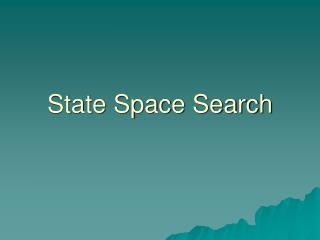Download PresentationState Space Search# State Space Search - PowerPoint PPT Presentation

Download Presentation##### State Space Search

Download Policy: Content on the Website is provided to you AS IS for your information and personal use and may not be sold / licensed / shared on other websites without getting consent from its author. While downloading, if for some reason you are not able to download a presentation, the publisher may have deleted the file from their server.

- - - - - - - - - - - - - - - - - - - - - - - - - - - E N D - - - - - - - - - - - - - - - - - - - - - - - - - - -
##### Presentation Transcript

1. State Space Search

2. State Space representation of a problem is a graph • Nodes correspond to problem states • Arcs correspond to steps in a solution process • One node corresponds to an initial state • One node corresponds to a goal state

3. Solution Path An ordered sequence of nodes from the initial state to the goal state

4. Search Algorithm Finds a solution path through a state space

5. The Water Jug Problem Suppose we have • An empty 4 gallon jug • An empty 3 gallon jug • A source of water • A task: put 2 gallons of water in the 4 gallon jug

6. Representation • State Space • Node on the graph is an ordered pair (x,y) • X is the contents of the 4 gallon jug • Y is the contents of the 3 gallon jug • Intitial State: (0,0) • Goal State: (2,N) N ε {0, 1, 2, 3}

7. Rules • if x < 4, fill x : (x,y)  (4,y) • if y < 3, fill y : (x,y)  (x,3) • if x > 0, empty x : (x,y)  (0,y) • if y > 0, empty y : (x,y)  (x,0) • if (x+y) >= 4 and y > 0 fill the 4 gallon jug from the 3 gallon jug (x,y)  (4, y – (4 – x)) • if (x+y) >= 3 and x > 0 Fill the 3 gallon jug from the 4 gallon jug (x,y)  (x –(3 – y), 3)) • if (x+y) <= 4 and y > 0 Pour the 3 gallon jug into the 4 gallon jug: (x,y)  (x+y), 0) • if (x+y) <= 3 and x > 0 pour the 4 gallon jug into the 3 gallon jug: (x,y)  (0, x + y)

8. Is there a solution path? Initial State: (0,0) Goal State: (2,N)

9. Breadth First Search (0,3) 1 2 (0,3) (4,0) (0,3) 6 7 2 (3,0) (4,3) (1,3) etc

10. Depth First (0,0) (4,0) 1 2 3 (4,3) 7 (0,3) (3,0) 2 (3,3) Etc. and without visiting already visited states

11. Backward/Forward Chaining Search can proceed • From data to goal • From goal to data Either could result in a successful search path, but one or the other might require examining more nodes depending on the circumstances

12. Data to goal is called forward chaining for data driven search Goal to data is called backward chaining or goal driven search

13. Examples • Water jug was data driven • Grandfather problem was goal driven • To make water jug goal driven: • Begin at (2,y) • Determine how many rules could produce this goal • Follow these rules backwards to the start state

14. Object • Reduce the size of the search space

15. Use Goal Driven • if • Goal is clearly stated • Many rules match the given facts • For example: the number of rules that conlude a given theorem is much smaller than the number that may be applied to the entire axiom set

16. Use Data Driven • If • Most data is given at the outset • Only a few ways to use the facts • Difficult to form an initial hypothesis • Water Jug: could go either way, but the presence of only a few rules, data existing in only a handful of states, and the difficulty of forming a hypothesis suggests data driven • Said another way: initial data constrains search